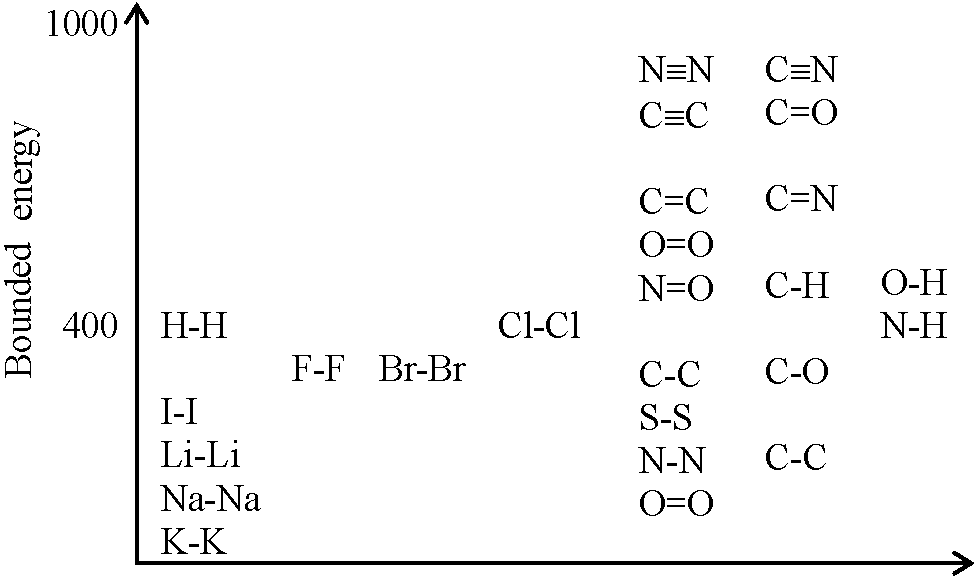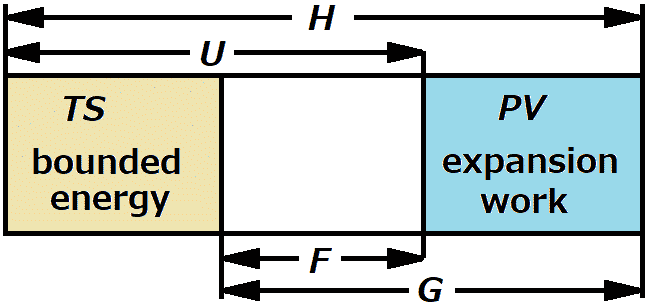(Upload on September 18 2023) [ 日本語 | English ]

## Thermodynamics (熱力学)

Mount Usu / Sarobetsu post-mined peatland
From left: Crater basin in 1986 and 2006. Cottongrass / Daylily

The study of the effects of work, heat and energy on a system

conserned with large scale observations without the consideration of molecular structure

Zeroth law: thermodynamic equilibrium and temperature
First law: work, heat, and energy
Second low: entropy
##### Symbols for buget change
Δ: finite change
δ: minutely-small change
d; instantaneous change

Ex. Q: heat quantity (specific heat) → ΔQ, δQ, dQ

#### History of thermodynamics (熱力学史)

Boyle, Robert 1627-1691
Mariotte, Edme 1620-1684
Becher, Johann Joachim 1635-1682
Huygens, Christiaan 1629-1695, Holland

1678 Huygens principle
1690 Huygens–Fresnel principle

Papin, Denis (1647-1712?), inventor in Frande

assistant of Huygens - in Engliand - assisting Boyle's experiments

[ temperature (climate)]

Savery, Thomas 1650-1715, England

developed steam engine

Newcomen, Thomas 1663-1729, England

developed practical steam engine

Lavoisier, Antoine Laurent 1743-1794
Stephenson, George 1781-1848, England, engineer

contributed to the commercialization of steam locomotives

Séguin, Marc 1786-1875, Frande: steam engine
Clapeyron, Emile 1799-1864, France: thermodynamics

## Heat engine (熱機関)

### First law of thermodynamics (熱力学の第1法則)

 = the law of conservation of energy ≡ the total energy of an isolated system is constant Mayer, Julius Robert von 1814-1878, Doctor in Germany one of the scientists who established the first law

### Reaction heat or heat of reaction (反応熱)

exothermic reaction (発熱反応) ⇔ endothermic reaction (吸熱反応)
##### Sorts of reaction heat
formation heat or heat of formation (生成熱), same as below
combustion heat (燃焼熱) - exothermic reaction

H2 + 1/2·O2 = H2O + 68.3 kcal

decomposition heat (分解熱)
neutralization heat (中和熱)
(dis)solution heat (溶解熱)

#### Bond energy (結合エネルギー)

Bond energy of C-H: (1661.7 KJ/mol)/4 = 415.4 KJ/mol### Enthalpy, H (エンタルピー)

Def. specific heat (比熱), C: the quantity of heat required to raise the temperature of 1 gram of a substance by 1°C (cal or J/g/°C

Water: 1 cal (or 4,186 J)/g/°C

Def. Enthalpy (heat content), HU + PV

a thermodynamic parameter that gauges the total heat present in a thermodynamic system where the pressure is regular
≡ the sum of the internal energy and the product of the pressure and volume of a thermodynamic system

Q. If the activation energy is equal for both forward and backward reactions, then (A. ΔH = 0)
Q. The change in enthalpy of a system is equivalent to the heat absorbed by the system at a (A. constant pressure)
Q. The change in enthalpy of an exothermic reaction is (A. always negative)
Q. If the heat is transmitted to a system at a steady pressure. In that case, the enthalpy of the system will (A. increase)
##### Factors affecting enthalpy
Amount of reactant and product
Physical state of reactants and products
Allotropic modification
Temperature and pressure
Def. Enthalpy change (of an atom): the amount of heat absorbed or evolved in a reaction carried out at a steady pressure
Def. Standard enthalpy of formation (標準生成エンタルピー), ΔfH°:

the change in enthalpy when 1 mole of the compound is formed under standard conditions

Q. Calculate the enthalpy change: CH4(g) + 2O2(g) → CO2(g) + 2H2O(l),

given that enthalpies of formation of CH4, CO2 and H2O are 74.8 kJ/mol, −393.5 kJ/mol, and −286 kJ/mol, respectively.

A. Enthalpy change:

ΔH°p – ΔH°r (p: products. r: reactants)
= (ΔH°CO2 + ΔH°H2O) – (ΔH°CH4 + 2ΔH°O2)
= (-393.5 − 286) – (74.8 + 2 × 0) (kJ/mol) = -890.7 kJ/mol

## Energy (エネルギー, E)

#### (Thermodynamic) free energy (自由エネルギー)

Helmholtz free energy, F (or A) = U - TS
Eq. U = -T2·/∂T(F/T)
Pr. RS = -T2·/∂T(F/T) = -T2·((T·∂F/∂T - dT/dT·F)/T2)

= F - T·∂F/∂T = F - T·((U - TS)/∂T) = F + TS = LS //

Gibbs free energy, GH - TS

[unit]

### Temperature (温度)

##### Celsius (摂氏)
Celsius (temperature) scale, or degree Celsius
t (°C) = T (K) - 273.16 (K)
##### Fahrenheit (華氏)
Fahrenheit (temperature) scale, or degree Fahrenheit
°F = 1.8 (= 9/5) × °C + 32 ⇔ °C = (5/9)·(°F - 32)

°F: -40___0___50__100
°C: -40_-17.8__10__37.8

### Entropy, S (エントロピー)

 Def. a measurement of the energy in a system or process that is not available to do work Cf. the amount of order or lack of order in a system (sociology) 1824 Carnot principle (カルノー原理) Clausius, Rudolf 1850 proposed the term "entropy" (appeared in 1865) = establishment of thermodynamicsFig. Relationships between the parameters of thermodynamics, U, H, F, G, TS and PV.